## Heat Transfer Miscellaneous

#### Heat-Transfer

1. Two infinite parallel plates are placed at a certain distance apart. An infinite radiation shield is inserted between the plates without touching any of them to reduce heat exchange between the plates. Assume that the emissivities of plates and radiation shield are equal. The ratio of the net heat exchange between the plates with and without the shield is

1.  qwith shields = 1 qwithout shields n + 1

here, n = 1
 q = 1 qwithout shields 2

##### Correct Option: A

 qwith shields = 1 qwithout shields n + 1

here, n = 1
 q = 1 qwithout shields 2

1. Three sets of parallel plates LM, NR and PQ are given in Figures 1, 2 and 3. The view factor Fij is defined as the fraction of radiation leaving plate I that is intercepted by plate J. Assume that the val ues of FL M and FN R ar e 0.8 and 0.4, respectively. The value of FPQ (round off to one decimal place) is _____.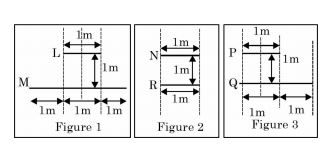1.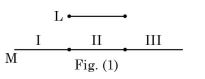FLM = F(I) + F(II) + F(III)
Where F is shape factor of surface area I, II and III of M with surface area of ‘L’
0.8 = F(I) + F(II) + F(III)
∵ (I) and (III) are symmetrical in shape so, shape factor of these surface will be same i.e. F(I) = F(III)
So, 0.8 = 2F(I) + F(II)... (1)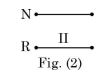In this area (II) is same as fig. (I) area II.
i.e. FNR = F(II) = 0.4... (2)
From equation (1),

 F(III) = 0.8 - 0.4 = 0.2 2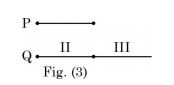Here, FPQ = F(II) + F(III)
= 0.4 + 0.2 = 0.6

##### Correct Option: BFLM = F(I) + F(II) + F(III)
Where F is shape factor of surface area I, II and III of M with surface area of ‘L’
0.8 = F(I) + F(II) + F(III)
∵ (I) and (III) are symmetrical in shape so, shape factor of these surface will be same i.e. F(I) = F(III)
So, 0.8 = 2F(I) + F(II)... (1)In this area (II) is same as fig. (I) area II.
i.e. FNR = F(II) = 0.4... (2)
From equation (1),

 F(III) = 0.8 - 0.4 = 0.2 2Here, FPQ = F(II) + F(III)
= 0.4 + 0.2 = 0.6

1. Sphere 1 with a diameter of 0.1 m is completely enclosed by another sphere 2 of diameter 0.4 m. The view factor F12 is

1.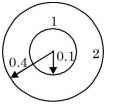F11 = 0
F12 = 1

##### Correct Option: DF11 = 0
F12 = 1

1. Consider the radiation heat exchange inside an annulus between two very long concentric cylinders. The radius of the outer cylinder is R0 and that of the inner cylinder is Ri. The radiation view factor of the outer cylinder onto itself is

1.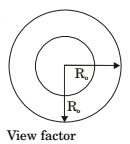F1– 1 = 0
F1– 2 = 1
Reciprocacy theorem
A2 F2– 1 = A1 F1– 2

 ⇒ F2– 1 = A1 F1– 2 A2

 = 2πRiL = Ri 2πRoL Ro

F2– 1 + F2– 2 = 1
 ∴ F2– 2 = 1 - F2– 1 = 1 - Ri Ro

##### Correct Option: DF1– 1 = 0
F1– 2 = 1
Reciprocacy theorem
A2 F2– 1 = A1 F1– 2

 ⇒ F2– 1 = A1 F1– 2 A2

 = 2πRiL = Ri 2πRoL Ro

F2– 1 + F2– 2 = 1
 ∴ F2– 2 = 1 - F2– 1 = 1 - Ri Ro

1. A solid sphere 1 of radius r is placed inside a hollow, closed hemispherical surface 2 of radius 4r. The shape factor F is,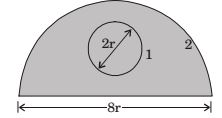1. f 11 + f 12 = 1
∴ f 12 = 1
f 21 A2 = f 12 A1

 ∴ f21 = f12A1 = 1 × 4πr2 = V = 1 A2 k (1 / 2)4π(4r)2 + π(4r)2 12

##### Correct Option: A

f 11 + f 12 = 1
∴ f 12 = 1
f 21 A2 = f 12 A1

 ∴ f21 = f12A1 = 1 × 4πr2 = V = 1 A2 k (1 / 2)4π(4r)2 + π(4r)2 12